# R – Charts and Graphs

R language is mostly used for the statistics and data analytics purpose to represent the data graphically in the software. To represent those data graphically, charts and graphs are used in R.
There are hundreds of charts and graphs present in R. For example, bar plot, box plot, mosaic plot, dot chart, coplot, histogram, pie chart, scatter graph, etc.

Below are the basic charts and graphs in R:

#### Bar Plot or Bar Chart

Bar plot or Bar Chart in R is used to represent the values in data vector as height of the bars. The data vector passed to the function is represented over y-axis of the graph. Bar chart can behave like histogram by using table() function instead of data vector.
Syntax:

```barplot(data, xlab, ylab)
```

where,

data is the data vector to be represented on y-axis
xlab is the label given to x-axis
ylab is the label given to y-axis

Note: To know about more optional parameters in `barplot()` function, use below command in R console:

```help("barplot")
```

Example:

 `# defining vector ` `x <``-` `c(``7``, ``15``, ``23``, ``12``, ``44``, ``56``, ``32``) ` ` `  `# output to be present as PNG file ` `png(``file` `=` `"barplot.png"``) ` ` `  `# plotting vector ` `barplot(x, xlab ``=` `"GeeksforGeeks Audience"``,  ` `           ``ylab ``=` `"Count"``, col ``=` `"white"``,  ` `           ``col.axis ``=` `"darkgreen"``,  ` `           ``col.lab ``=` `"darkgreen"``) ` ` `  `# saving the file ` `dev.off()  `

Output: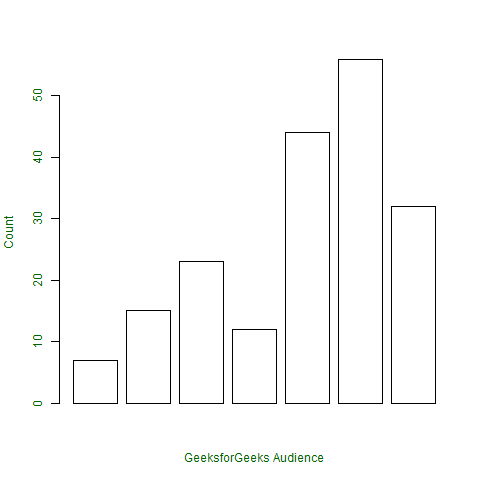#### Pie Diagram or Pie Chart

Pie chart is circular chart divided into different segments according to the ratio of data provided. The total value of pie is 100 and the segments tells the fraction of the whole pie. It is another method to represent statistical data in graphical form and `pie()` function is used to perform the same.
Syntax:

```pie(x, labels, col, main, radius)
```

where,

x is data vector
labels shows names given to slices
col fills the color in the slices as given parameter
main shows title name of the pie chart
radius indicates radius of the pie chart. It can be between -1 to +1

Note: To know about more optional parameters in `pie()` function, use below command in R console:

```help("pie")
```

Example:
Assume, vector x indicates the number of articles present on GeeksforGeeks portal in categories names(x)

 `# defining vector x with number of articles ` `x <``-` `c(``210``, ``450``, ``250``, ``100``, ``50``, ``90``) ` ` `  `# defining labels for each value in x ` `names(x) <``-` `c(``"Algo"``, ``"DS"``, ``"Java"``, ``"C"``, ``"C++"``, ``"Python"``) ` ` `  `# output to be present as PNG file ` `png(``file` `=` `"piechart.png"``) ` ` `  `# creating pie chart ` `pie(x, labels ``=` `names(x), col ``=` `"white"``,  ` `main ``=` `"Articles on GeeksforGeeks"``, radius ``=` `-``1``, ` `col.main ``=` `"darkgreen"``) ` ` `  `# saving the file ` `dev.off() `

Output: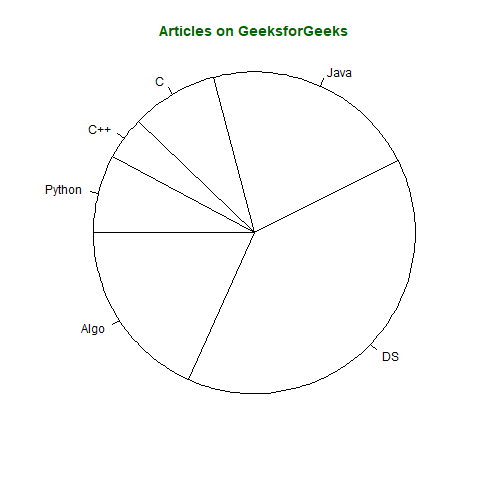Pie chart in 3D can also be created in R by using following syntax but requires `plotrix` library.
Syntax:

```pie3D(x, labels, radius, main)
```

Note: To know about more optional parameters in `pie3D()` function, use below command in R console:

```help("pie3D")
```

Example:

 `# importing library plotrix for pie3D() ` `library(plotrix) ` `# defining vector x with number of articles ` `x <``-` `c(``210``, ``450``, ``250``, ``100``, ``50``, ``90``) ` ` `  `# defining labels for each value in x ` `names(x) <``-` `c(``"Algo"``, ``"DS"``, ``"Java"``, ``"C"``, ``"C++"``, ``"Python"``) ` ` `  `# output to be present as PNG file ` `png(``file` `=` `"piechart3d.png"``) ` ` `  `# creating pie chart ` `pie3D(x, labels ``=` `names(x), col ``=` `"white"``,  ` `main ``=` `"Articles on GeeksforGeeks"``, ` `labelcol ``=` `"darkgreen"``, col.main ``=` `"darkgreen"``) ` ` `  `# saving the file ` `dev.off() `

Output: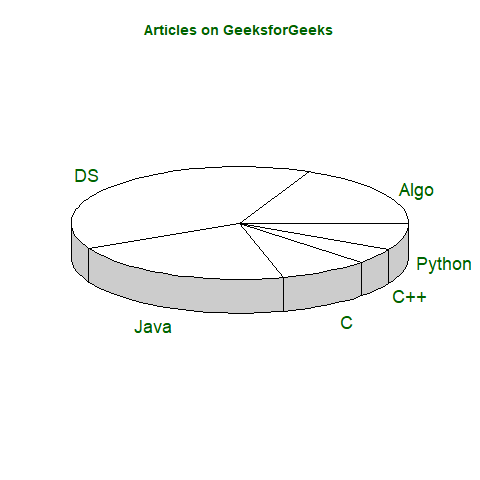#### Histogram

Histogram is a graphical representation used to create a graph with bars representing the frequency of grouped data in vector. Histogram is same as bar chart but only difference between them is histogram represents frequency of grouped data rather than data itself.

Syntax:

```hist(x, col, border, main, xlab, ylab)
```

where,

x is data vector
col specifies the color of the bars to be filled
border specifies the color of border of bars
main specifies the title name of histogram
xlab specifies the x-axis label
ylab specifies the y-axis label

Note: To know about more optional parameters in `hist()` function, use below command in R console:

```help("hist")
```

Example:

 `# defining vector ` `x <``-` `c(``21``, ``23``, ``56``, ``90``, ``20``, ``7``, ``94``, ``12``,  ` `       ``57``, ``76``, ``69``, ``45``, ``34``, ``32``, ``49``, ``55``, ``57``) ` `  `  `# output to be present as PNG file ` `png(``file` `=` `"hist.png"``) ` `  `  `# hist(x, main = "Histogram of Vector x",  ` `          ``xlab ``=` `"Values"``,  ` `          ``col.lab ``=` `"darkgreen"``,  ` `          ``col.main ``=` `"darkgreen"``) ` `  `  `# saving the file ` `dev.off() `

Output: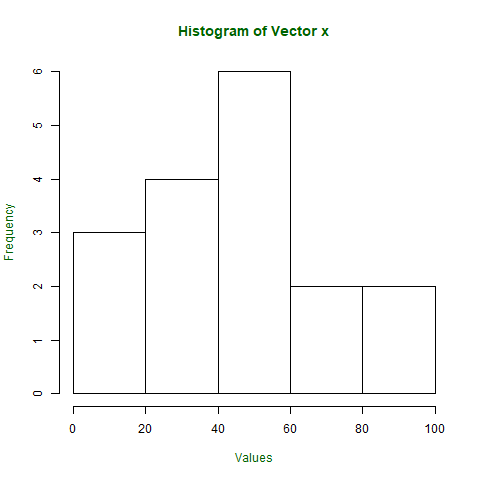#### Scatter Plot

Scatter plot is another type of graphical representation used to plot the points to show relationship between two data vectors. One of the data vector is represented on x-axis and another on y-axis.
Syntax:

```plot(x, y, type, xlab, ylab, main)
```

Where,

x is the data vector represented on x-axis
y is the data vector represented on y-axis
type specifies the type of plot to be drawn. For example, “l” for lines, “p” for points, “s” for stair steps, etc.
xlab specifies the label for x-axis
ylab specifies the label for y-axis
main specifies the title name of the graph

Note: To know about more optional parameters in `plot()` function, use below command in R console:

```help("plot")
```

Example:

 `# taking input from dataset Orange already present in R ` `orange <``-` `Orange[, c(``'age'``, ``'circumference'``)] ` ` `  `# output to be present as PNG file ` `png(``file` `=` `"plot.png"``) ` ` `  `# plotting ` `plot(x ``=` `orange\$age, y ``=` `orange\$circumference, xlab ``=` `"Age"``, ` `ylab ``=` `"Circumference"``, main ``=` `"Age VS Circumference"``, ` `col.lab ``=` `"darkgreen"``, col.main ``=` `"darkgreen"``, ` `col.axis ``=` `"darkgreen"``) ` ` `  `# saving the file ` `dev.off() `

Output: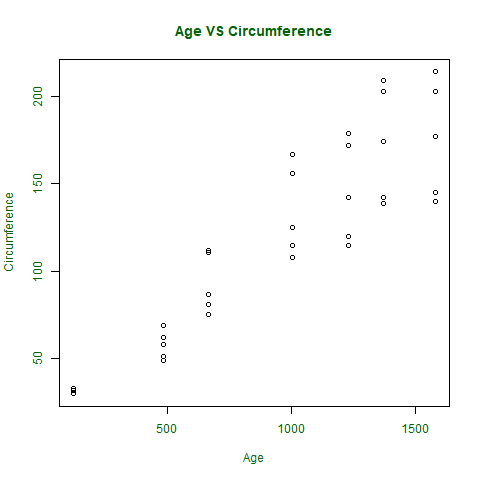If a scatter plot has to be drawn to show relation between 2 or more vectors or to plot the scatter plot matrix between the vectors, then `pairs()` function is used to satisfy the criteria.
Syntax for basic use of `pairs()` function

```pairs(~formula, data)
```

where,

~formula is the mathematical formula such as ~a+b+c
data is the dataset form where data is taken in formula

Note: To know about more optional parameters in pairs() function, use below command in R console:

```help("pairs")
```

Example :

 `# output to be present as PNG file ` `png(``file` `=` `"plotmatrix.png"``) ` ` `  `# plotting scatterplot matrix  ` `# using dataset Orange ` `pairs(~age ``+` `circumference, data ``=` `Orange,  ` `col.axis ``=` `"darkgreen"``) ` ` `  `# saving the file ` `dev.off() `

Output: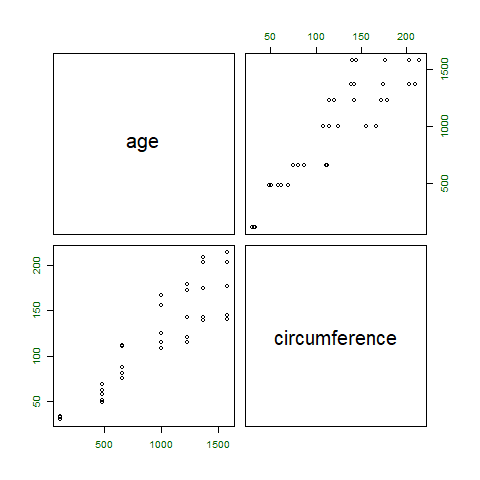#### Box Plot

Box plot shows how the data is distributed in the data vector. It represents five values in the graph i.e., minimum, first quartile, second quartile(median), third quartile, the maximum value of the data vector.

Syntax:

```boxplot(x, xlab, ylab, notch)
```

where,

x specifies the data vector
xlab specifies the label for x-axis
ylab specifies the label for y-axis
notch, if TRUE then creates notch on both the sides of the box

Note: To know about more optional parameters in `boxplot()` function, use below command in R console:

```help("boxplot")
```

Example:

 `# defining vector with ages of employees ` `x <``-` `c(``42``, ``21``, ``22``, ``24``, ``25``, ``30``, ``29``, ``22``, ` `       ``23``, ``23``, ``24``, ``28``, ``32``, ``45``, ``39``, ``40``) ` ` `  `# output to be present as PNG file ` `png(``file` `=` `"boxplot.png"``) ` ` `  `# plotting ` `boxplot(x, xlab ``=` `"Box Plot"``, ylab ``=` `"Age"``, ` `col.axis ``=` `"darkgreen"``, col.lab ``=` `"darkgreen"``) ` ` `  `# saving the file ` `dev.off() `

Output: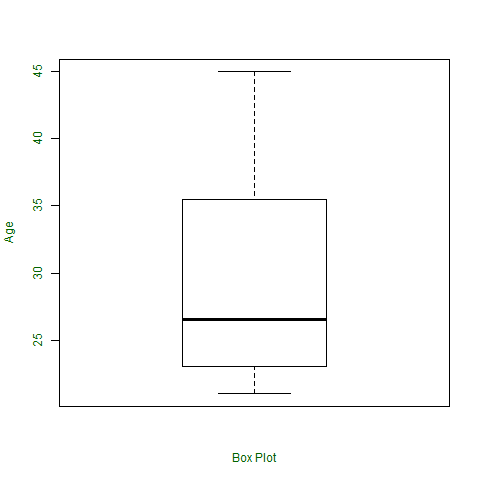My Personal Notes arrow_drop_upCheck out this Author's contributed articles.

If you like GeeksforGeeks and would like to contribute, you can also write an article using contribute.geeksforgeeks.org or mail your article to contribute@geeksforgeeks.org. See your article appearing on the GeeksforGeeks main page and help other Geeks.

Please Improve this article if you find anything incorrect by clicking on the "Improve Article" button below.

Article Tags :

2

Please write to us at contribute@geeksforgeeks.org to report any issue with the above content.